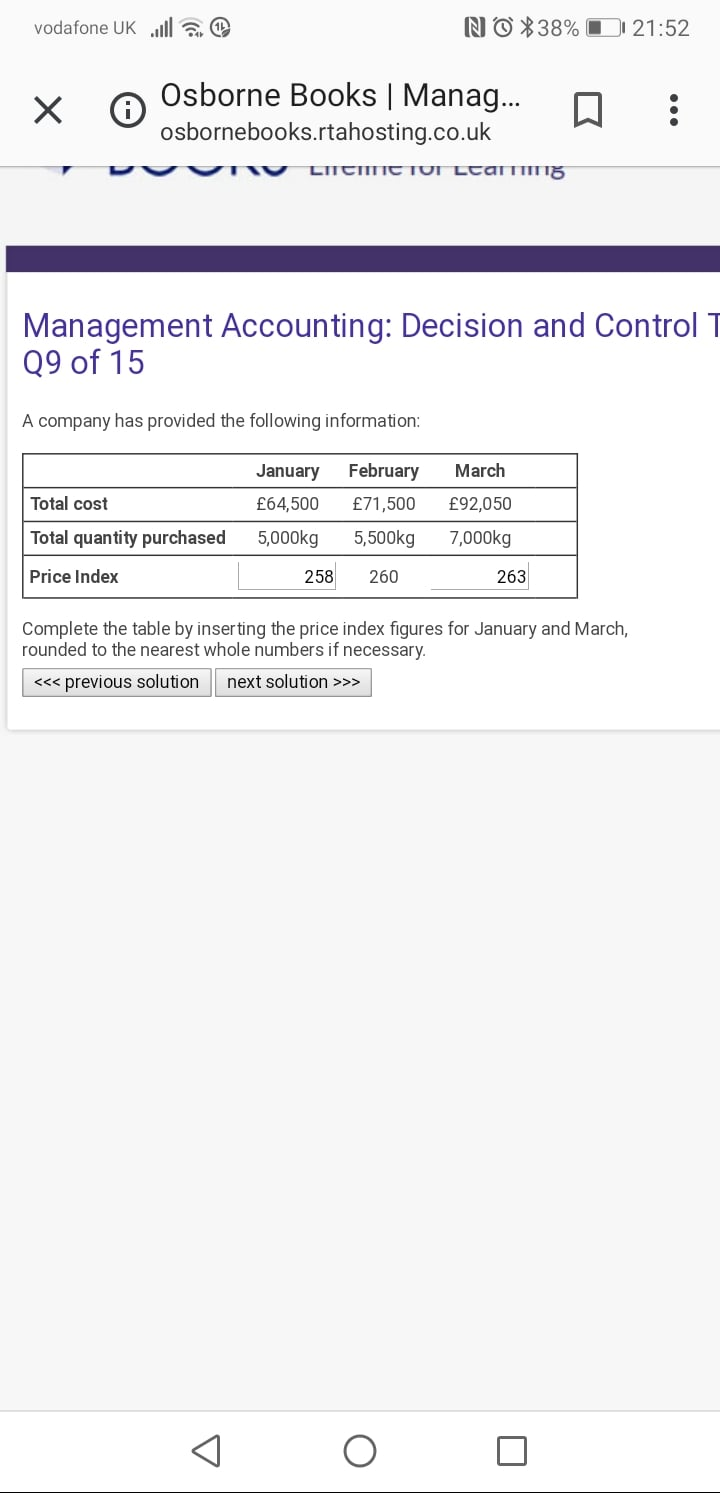# Index calculation

Can anyone help with how the index is calculated for January and March for the attached? Thank you.• logically this would be my answer: calculate the cost of each item first for each month then do the indexes:

J: 64,500/5000 = £12.9
F: 71500/5500= £14.30
M: 92,050/7000 = £13.15

14.30 = 260
14.30:260=12.90:x
x = 12.90x260/14.30 = 234
etc

• SilvaAat said:

Thank you

where did you get that numbers from? 258 and 263?
• @SilvaAat

64500/5000 = 12.90
71500/550 = 13
92050/7000 = 13.15

12.90/13*260=258
13.15/13*260=263

@fedemenni those answers are given as the solution in the Osbourne quiz.....but they don't actually tell you how they got the answers......
• ok, I actually did the calculation correct but the result was incorrect - i think i just entered the wrong amounts on the calculator @Dimps92